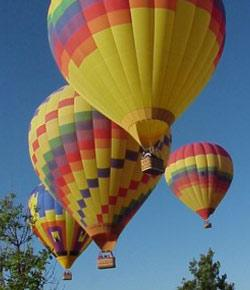# Gas Laws: Boyle's And Charles' Law

Approved & Edited by ProProfs Editorial Team
At ProProfs Quizzes, our dedicated in-house team of experts takes pride in their work. With a sharp eye for detail, they meticulously review each quiz. This ensures that every quiz, taken by over 100 million users, meets our standards of accuracy, clarity, and engagement.
| Written by Kwchiro
K
Kwchiro
Community Contributor
Total Contribution - 40 | Total attempts - 312,648
Questions: 10 | Attempts: 8,416Settings.

• 1.

### A balloon occupies a volume of 2.0 L at 40oC. How much volume will it occupy at 30oC?

• A.

1.5 L

• B.

1.94 L

• C.

1.6 L

B. 1.94 L
Explanation
Make sure you change to Kelvin!! (add 273) or you will get the WRONG answer!!
V1/T1 = V2/T2 Charles' law

Rate this question:

• 2.

### What is the final pressure in a balloon when the volume is changed from 1.5  L to a volume of 2.5L at constant temperature? (Initial pressure is 1.1 atm).

• A.

.66 atm

• B.

.66 kPa

• C.

4.125 atm

A. .66 atm
Explanation
Boyle's Law: (P1) (V1) = (P2) (V2)
The units will be the SAME on both sides of the equation.

Rate this question:

• 3.

### Gas in a balloon occupies 3.3  L. What volume will it occupy if the pressure is changed from 100 kPa to 90  kPa (at constant temperature of 310 K (about room temperature).

• A.

29,700 L

• B.

2.97 L

• C.

3.6 L

C. 3.6 L
Explanation
Boyle's Law: (P1) (V1) = (P2) (V2)
The units will be the SAME on both sides of the equation.

Rate this question:

• 4.

### What is the final pressure in a vessel when the volume is changed from 1.3  L to a volume of 1.55 L at constant temperature? (Initial pressure is 1.5 atm).

• A.

1.25 L

• B.

1.65 L

• C.

1.49 L

A. 1.25 L
Explanation
Boyle's Law: (P1) (V1) = (P2) (V2)
The units will be the SAME on both sides of the equation.

Rate this question:

• 5.

### A balloon occupies 3.2 L at 37oC. How much will it occupy at 42oC?

• A.

3.15 L

• B.

3.25 L

• C.

312,480 L

B. 3.25 L
Explanation
Make sure you change to Kelvin!! (add 273) or you will get the WRONG answer!!
V1/T1 = V2/T2 Charles' law

Rate this question:

• 6.

### A gas occupies a balloon with a volume 2.0 L at 33oC. How much volume will it occupy at 37oC, if pressure remains constant.

• A.

1.97 L

• B.

186,000 L

• C.

2.06 L

C. 2.06 L
Explanation
Make sure you change to Kelvin!! (add 273) or you will get the WRONG answer!!
V1/T1 = V2/T2 Charles' law

Rate this question:

• 7.

### A gas expands from a volume 2.0 L at 36oC to a volume of 2.5 L, what is the final temperature, if the pressure is constant?

• A.

386 K ( which equals 113.25 Celsius)

• B.

247.2 K (which equals -25.8 C)

• C.

61.8 K (which equals -211.2 C)

A. 386 K ( which equals 113.25 Celsius)
Explanation
Make sure you change to Kelvin!! (add 273) or you will get the WRONG answer!!
V1/T1 = V2/T2 Charles' law

Rate this question:

• 8.

### If the air inside a balloon is heated, the volume will:

• A.

Increase

• B.

Decrease

• C.

Increase a little, then decrease.

A. Increase
Explanation
Temperature and Volume are directly related (Charles' law): When temperature is increased, volume will also increase.

Rate this question:

• 9.

### The kinetic theory of gases, which of the following is important for an ideal gas:

• A.

There is no attraction or repulsion between the gas molecules, whereas there IS attraction between molecules of liquids or solids.

• B.

The distance between molecules in ideal gases is closer than in liquids or solids.

• C.

The gases are NOT fluid

A. There is no attraction or repulsion between the gas molecules, whereas there IS attraction between molecules of liquids or solids.
Explanation
The correct answer is that there is no attraction or repulsion between the gas molecules, whereas there IS attraction between molecules of liquids or solids. This is important for an ideal gas because it assumes that the gas molecules do not interact with each other, except during collisions. This allows for simplifications in the mathematical models used to describe the behavior of ideal gases. In contrast, liquids and solids have intermolecular forces that result in attractions between their molecules, leading to different behaviors and properties.

Rate this question:

• 10.

### Which of the following describes the relationship between P and V?

• A.

Directly proportional

• B.

Inversely proportional

• C.

UnrelatedBack to top